Using Mathematica to Correct Flaws in Textbook Models for Derivatives Trading

Back to this customer story

From the first chapter of Modelling Financial Derivatives with Mathematica, by William Shaw

One of the very few complete solutions to this list of requirements is the Mathematica system. Originally subtitled "A System for Doing Mathematics by Computer", it is uniquely able as a derivatives modelling tool.

Chapter 1. Advanced Tools for Rocket Science

1.1 Why You Should Use Mathematica for Derivatives Modelling

When expressed in mathematical terms, the modelling of a derivative security amounts to understanding the behaviour of a function of several variables in considerable detail. We need to know not just how to work out the function (the value of an option), but also how to secure an appreciation of the sensitivity of the value to changes in any of the parameters of the function. Such an appreciation is most reliable when we have both a view of the local sensitivities, expressed through partial derivatives, and a global view, expressed by graphical means. The partial derivatives, expressed in financial terms, are the "Greeks" of the option value, and may be passive sensitivity variables, or may be active hedging parameters. Such option valuations may, in simpler cases, be based on analytical closed forms involving special functions, or, failing that, may require intensive numerical computation requiring some extensive programming.

If one was asked afresh what sort of a system would combine together the ability to:

i) deal with a myriad of special functions, and do symbolic calculus with them;
iii) allow complex structures to be programmed;
iv) visualize functions in two, three or more dimensions;

and to do so on a range of computer platforms, one would come up with a very short list of modelling systems. Neither spreadsheets nor C/C++ would feature on the list, due to their fundamental failure to cope with point (i)--symbolic algebra and calculus.

One of the very few complete solutions to this list of requirements is the Mathematica system. Originally subtitled "A System for Doing Mathematics by Computer", it is uniquely able as a derivatives modelling tool. In particular, version 3.0 and later includes substantial new numerical functionality, and it is capable of efficient compiled numerical modelling on large structures. Mathematica can manage not just scalar, vector and matrix data, but also tensors of arbitrary rank; and its performance scales well to large and realistic problems. This book is, in part, an exploration of these capabilities. It is also an exploration of valuation algorithms and what can go wrong with them.

Exploring the Fundamentals with Mathematica

This book is not just an exploration of a few well-known models. We shall also explore the matter of solving the Black-Scholes differential equation in a Mathematica-assisted fashion. We can explore:

i) Separation of Variables;
ii) Similarity Methods;
iii) Green's Functions and Images;
iv) Finite-Difference Numerics;
v) Tree Numerics;
vi) Monte Carlo Simulation

as solution techniques, all within the Mathematica environment. A comparison of these approaches and their outcomes is more than just an interesting academic exercise, as we shall consider next.

Informal Definitions of Model and Algorithm Risk

Model Risk can arise in a variety of ways. Given a financial instrument, we generally formulate a three-stage valuation process:

a) Conceptual description;
b) Mathematical formulation of the conceptual description;
c) Solution of the mathematical model through an analytical and/or numerical process.

It is possible to make mistakes, or perhaps unreasonable simplifying assumptions, at any one of these three stages. Each of these gives rise to a form of model risk--the risk that the process from reading a contract to responding with a set of answers (fair value, delta, gamma, ..., implied volatility) has led to the wrong answer being supplied.

This book uses Mathematica to look intelligently at the third point, which we might call algorithm risk. One might think that this can be eliminated just by being careful--the real story is that derivative securities are capable of exhibiting some diverse forms of mathematical pathology that confound our intuition and play havoc with standard or even state-of-the-art algorithms. This book will present some familiar and rather less familiar examples of trouble.

I wish to emphasize that this is not just a matter of worrying about a level of accuracy that is below the "noise level" of traders. It is possible to get horribly wrong answers even when one thinks that one is being rather careful. I also tend to think that it is the job of a Quants Team to supply as accurate an answer as possible to trading and sales teams. If they want to subtract or add 50 basis points to your answer, for whatever reasons, that is their responsibility!

Verification and Resolving Disagreement Amongst Experts

A good rule of thumb is that in general you can give what you think is the same problem to six different groups and obtain at least three materially different answers. In derivatives modelling you can usually get four different answers, especially if you include:

a) One Analytics fanatic;
b) One Tree-Model fanatic;
c) One Monte-Carlo fanatic;
d) One Finite-Difference fanatic

among the group of people you choose. I use the term "fanatic" quite deliberately. I once had a party where I was told categorically that "you absolutely had to use Martingales--these PDE people have got it all wrong", and I know of at least one PDE book that makes almost no reference to simulation, and one excellent simulation book that went so far as to describe the PDE approach as "notorious". In fact, all these methods should give the same answer when applied carefully to a problem to which they can all be applied. You can get small but irritating non-material differences out of models because of differences of view over things like calendar management, units, continuously compounded versus annual stuff, and so on. That sort of thing can and should generally be resolved by getting the Risk Management team to bash heads together. The issues arising from genuine differences between models of a different type, and more worringly still, between models of a similar type, require a much more disciplined approach.

The only way to sort out material differences in answers is to perform some form of verification study. This can be done in various ways, of which the two most important ones are:

a) Comparison of modelling systems with some form of absolutely accurate benchmark (typically only available for simpler models, but we must check that complex algorithms do work correctly when specialized to simple cases);

b) Intercomparison of models and codes when applied to complex instruments.

The basic idea is to define a comprehensive set of test problems, and run off a benchmark model and operational systems against as many others as are available.

In other fields of study this is actually a common process. I hope this text encourages similar efforts within the derivatives modelling community.

Resource Management

The essential requirements of such an approach include duplication of effort. My view is that no number should be believed unless it can be justified as the result of at least two different modelling efforts (or at least that the same system has been through such an intercomparison or benchmarking study in the past, and that it has been documented). This is generally not what management involved in resource allocation want to hear! The point is that quality is only achieved through careful and critical testing and appraisal.

1.3 The Power of Symbolic Computer Calculus

In recent years a number of tools have emerged that extend the utility of computers from "number crunching" to the domain of carrying out algebra and calculus. More mature systems combine such symbolic capability with advanced numerical algorithms, 2D, 3D, and animated graphics, and a programming environment. Such tools are particularly relevant to the derivatives modelling and testing area due to

a) the fact that they have built-in routines for most basic common operations;
b) the ability to do calculus with simple and complex functions can eliminate many of the headaches of computing Greeks;
c) the ability to compare analytical symbolic models directly with the results from a numerical algorithm;
d) the ability to visualize the results, and literally see the errors if there are any.

It is my firm belief that in the long term such hybrid symbolic-numeric-graphical systems will replace the spreadsheet and languages such as C or C++ for derivatives modelling. Their primary advantage lies in the provision of advanced symbolic capabilities. In a numerically based system the only two ways to differentiate to get a Greek such as delta, are a) to work out the derivative on paper and code it up, b) work out the value of an option for one or two neighbouring values of the parameter, and take differences. Both of these have drawbacks, and the potential for human error at the pen-and-paper stage, and numerical errors arising from numerical differentiation problems. Instead we can now ask the computer to do the differentiation for us. Such symbolic environments, rich as they are in special-function libraries, can dramatically reduce development time below that for spreadsheet or C/C++ systems. Furthermore, in the testing and verification context, they offer a simple integrated environment where a numerical model can be compared directly with its exact form. And I mean exact--current systems can do arbitrary-precision arithmetic with ease, and can do so with complicated "special functions". The latest versions of these systems, as is the case with Mathematica 3.0 or later, can also produce mathematical typeset material, so you can write your code, write a mathematical model, test them, plot the results, and produce a report for risk management/trading/regulatory bodies--all within one environment.

There are many problems with spreadsheet environments. An extensive discussion of the issues is given by R. Miller (1990) in Chapter 1 of his text, Computer-Aided Financial Analysis, where he outlines several principles to which financial modelling environments should conform, and explains why spreadsheets fail to meet them. My own simplistic interpretation of Miller's views (i.e., my own prejudice) is that spreadsheets are the best way yet invented of muddling up input data, models, and output data. More seriously, their fabled capability for doing "what if" calculations is at once both erroneous and misleading. In the particular context of derivatives modelling, the modelling of Greeks within spreadsheets by revaluing for neighbouring values of the parameters is an abomination. The point is that you should use exact calculus wherever possible to extract partial derivatives. As Miller points out, the "what-if" concept is also limited to numerical variations. We want a system where we can also vary structural properties ("what if American rather than European"). This leads rather naturally to the desire for an object-oriented approach.

There is one virtue of the spreadsheet environment, and that is the tabular user interface. There is no doubt that for instruments requiring a small number of input and output parameters, such a table of data for several such instruments is extremely useful. However, even the interface virtues of spreadsheets are strained by the complexities of instruments such as convertible bonds. The information relevant to one such bond can easily require several interlinked sheets.

Flushing POO Away?

Not for nothing, in my view, is the Spanish acronym for object-oriented programming POO, rather than OOP. C++ has become entrenched as a modelling system in many finance houses. In the view of the author, the job of a "Quant" is to analyse, test, and price financial instruments as quickly and reliably as possible to respond to trading needs. There can be no greater waste of a Quant's time than having to write and debug algorithms in C++, let alone, God forbid, waste further energy embedding them in a spreadsheet. If one needs to do a highly numerically intensive model for which a system such as Mathematica may, at least for the present, be inadequate, the solution, at least for now, is to use a system designed for the task, such as highly optimized FORTRAN 90. Objections to Mathematica based on speed of execution are now essentially irrelevant due to improvements in low-price high-speed CPUs, and the increased efficiency of the internal compiled numerical environment in Mathematica. C++ systems are much slower to write, slow to compile, and their execution time advantage is now marginal. The great dream of system managers is that once the headaches of building a library of core C++ library routines is complete, modellers can build new algorithms quickly. Sadly, this is only a dream. OOP projects mysteriously seem to require massive increases in headcounts and budgets, yet are nevertheless the beloved of system managers.

This is NOT to say that an object-oriented approach is a bad idea--the problem is getting the dream back on track. My view is that the base-line system for object-oriented derivatives development should be at the level of a system such as Mathematica, where there is a symbolic capability, and already a huge collection of basic objects already in place with their mathematics sorted out. A good question to ask an OOP-based derivative team is how much time they have wasted coding up interpolation, Normal distributions, Greeks, and all kinds of special functions and capabilities that already exist in Mathematica. In this text I have not attempted a complete object-oriented approach. My focus has been on making sure that the answers are correct. I leave it to others to adopt some of these methods and algorithms into a more structured environment.

The Use of Mathematica

The power of Mathematica as an investigate tool becomes clear in this context. We can define a series of test problems and use the special function capabilities of Mathematica to characterize the solution exactly, using Mathematica's infinite-precision arithmetic to get the results with as much accuracy as we desire. Next we use Mathematica's symbolic calculus capabilities to define the Greeks by differentiation. Then, for more complex models, we build tree, Monte-Carlo and Finite-Difference models within Mathematica. Then we make a comparison, within Mathematica, between these analytical forms and the numerical solutions, and the solutions from other company systems. The results are compared, and the reasons for any differences or other problems are explored and resolved.

A Challenge!

To those who find my comments on traditional modelling systems extreme or ill-informed, I issue this challenge. There is now available an exact solution for the continuously averaged arithmetic Asian option. This is the Geman-Yor model described in Chapter 10. I got this up and running in Mathematica, and tested it, in a total of six hours. To anyone who can implement this in C++, and get answers accurate to 4dp, I offer a bottle of quality vintage champagne. If they can do it in less than 40 hours of coding time, two bottles. To anyone who can get it working as accurately in a spreadsheet alone, I offer a case of good red wine, however long it takes.

1.4 A Case Study in Chaos--Does Implied Volatility Make Any Sense?

As an illustration of something that is easy to explore in a system such as Mathematica, we give an example of how one can investigate a topic within a system combining symbolic models and graphics. The topic chosen is "Implied Volatility", and the point is to make it clear just how infrequently this concept makes any sense. This is important from both a mathematical point of view, as we need to note when a function cannot be inverted as it is "many to one", and from a trading point of view--implied volatility is often used as a key parameter.

Traders are persistent in asking for implied volatilities. You can give them a number, but it might not make any sense at all. Or rather, you can feed a market price for an instrument to a system which does all sorts of fancy things to find the volatility consistent with the market price given all the other data, and this volatility can make no sense at all. It is a good idea to list the nice situation and all the horrors that can arise. I emphasize that these are not issues linked to a particular solution method, such as Newton-Raphson or bisection, where particular problems linked to the solution method may arise. These are matters of principle that will cause trouble whatever solution technique is employed.

Things Are OK

When the valuation is a monotonic function of volatility (strictly increasing or decreasing), there is usually no problem. The simple case of a vanilla European call option, as developed by Black and Scholes (1973) falls into this category. Let's build a symbolic model of this option and plot the value as a function of volatility--we take this opportunity to show how easy it is to write down a model in a symbolic system such as Mathematica.We plot the option value as a function of the volatility, for an option where the strike is at 10, the underlying at 11, for a vanilla Euro-call with one year to expiry, 5% risk-free rate (continuously compounded) and zero dividends.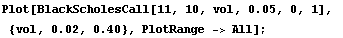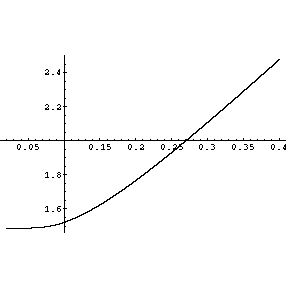We can also build an implied volatility calculator in a moment (FindRoot is a combination Newton-Raphson/Secant solver).

BlackScholesCallImpVol[s_,k_,r_,q_,t_,price_] :3Dsd /. FindRoot[BlackScholesCall[s,k, sd, r, q, t]3D3Dprice,{sd,0.2}];

Because the function is strictly increasing, we get a one-one mapping between prices and volatilities.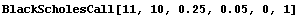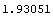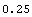The results are stable--a small change in the market price causes a small shift in the implied volatilities.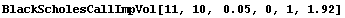Things Are Sometimes OK, Sometimes Highly Unstable

It does not take much to mess up the calculation of implied volatility. Let's add a dilution effect to the Black-Scholes model, providing a simple model for a warrant. This is one of the simpler warrant-pricing models discussed by Lauterbach and Schultz (1990).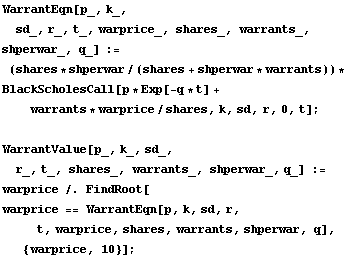When we plot the result for price against volatility, we see that there is a nice monotonic region for larger volatilities, but that the curve becomes very flat as the volatility dips below 20%. The corresponding inversion for the implied volatility then becomes either unstable or impossible.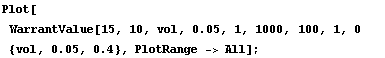Things Are Definitely not OK--There Are Two or Even Three Implied Volatilities

You do not have to make the option that much more complicated to really get in a mess. A simple barrier option will exhibit the phenomenon of there being two volatilities consistent with a given valuation. Suppose we consider an up and out call where the underlying is at 45, the strike at 50, and the knockout barrier at 60. As we first increase the volatility from a very low value, the option value increases. Then, as the volatility increases further, the probability of knockout increases, lowering the value of the option. By tweaking in a rebate we can arrange for there to be three solutions for a small range of market option prices. The model of barriers we use is the analytical model developed by Rubenstein and Reiner, 1991--this has again been built in Mathematica, but we suppress the details of the symbolic model here--it is given in Chapter 8. The two graphs following show cases where there are two and three implied volatilities, respectively.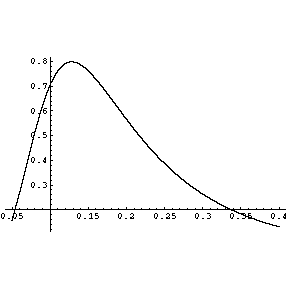In other words, vega is positive apart from an interval in which it is negative.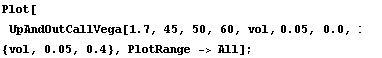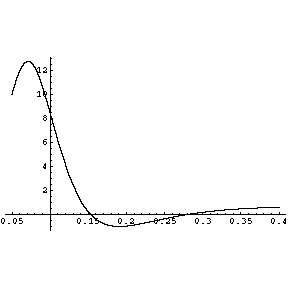In Chapter 8 you will see how vega is defined by elementary differentiation using Mathematica's "D" operator.

Things Are Impossible--There Are Infinitely Many Answers

If you want to see just how bad it can get, a barrier option can do still more interesting things. If we consider an Up and Out Put, where the strike coincides with the barrier, and arrange for the risk-free rate and the dividend yield to coincide, we can get a dead zero vega (and zero gamma too). So if the market price happens to coincide with the computed value, you can have any implied volatility you want. Otherwise there is no implied volatility.

Plot3D[UpAndOutPut[0, S, 50, 50, vol,0.1, 0.1, 1],
{S, 30, 50}, {vol, 0.05, 0.4 }, PlotRange -> {-0.01, 0.01}, PlotPoints -> 40];You should be grateful that this is all that goes wrong. Fortunately derivatives modellers tend to use real numbers. In complex function theory, Newton-Raphson and other iterative solution techniques are famous for their generation of fractals and chaotic behaviour. But it is a good idea to avoid quoting implied volatilities unless you have a firm grasp on the characteristics of an instrument. Only the very simplest options behave well, in this respect. More generally, you should not report implied volatility results unless you have first checked that the absolute value of vega is bounded away from zero. Otherwise the implied volatility is at best unstable and possibly undefined--in such cases it should not be reported.

Remarks

This little study has illustrated several points about the use of Mathematica:

a) You can build symbolic models very quickly;
b) Greeks can be investigated by symbolic differentiation;
c) We can visualize our models using two- and three-dimensional graphics;
d) We can expose peculiar situations with relative ease.

Later in this book we shall see how the exposure of significant problems can be extended to intensively numerical processes.

For now we return to the fundamentals. In the next three chapters we lay the foundations of

a) Using Mathematica;
b) Financial mathematics of derivatives pricing;
c) The mathematics for solving partial differential equations (the Black-Scholes PDE).

A Final Note

We live in a world where applied quantitative science in general, and financial modelling in particular, is increasingly dominated by purely numerical modelling. The view of the author is that the analytical approach is being inappropriately eclipsed by a rather mindless approach involving flinging models at a computer and seeing what numbers come out.

This is not the best way to use computers. We are seeking insight into the behaviour of complex systems, and this is best obtained by first attempting an analytical treatment. This first focuses our instincts, and gives us test cases to check against when we finally come to adopt a purely numerical approach. Symbolic algebra systems such as Mathematica allow us to extend the analytical approach to much more complicated systems. The numerical approach should always be the method of absolute last resort, and should then be checked extensively against the analytical results. This is the guiding principle beyond the approach taken by this text.

Chapter 1 Bibliography

Black, F. and Scholes, M., Journal of Political Economy, Vol. 81, p. 637, 1973. (Reprinted and widely accessible as Chapter 1 of "Vasicek and Beyond", ed. Hughston, Risk Publications, 1996).

Lauterbach, B. and Schultz, P., Journal of Finance, XLV, 4, p. 1181, September 1990.

Miller, R.M. , Computer-Aided Financial Analysis, Addison-Wesley, 1990.

Rubenstein, M. and Reiner, E. Breaking Down the Barriers, RISK Magazine, September 1991.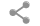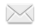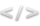Share Email Embed

Related Stories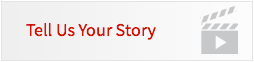Get started with Wolfram technologies, or work with us to apply computational expertise to your projects.

Questions? Comments? Get in touch: 1-800-WOLFRAM, or email us »